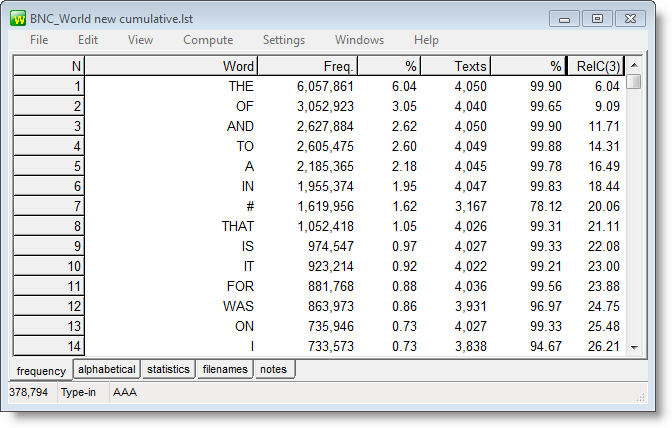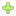# WordSmith Tools Manual

 Navigation: Controller > columns of data Compute ()

The point of it…

This function brings up a calculator, where you can choose functions to calculate values which interest you. For example, a word list routinely provides the frequency of each type, and that frequency as a percentage of the overall text tokens. You might want to insert a further column showing the frequency as a percentage of the number of word types, or a column showing the frequency as a percentage of the number of text files from which the word list was created.This word-list has a column which computes the cumulative scores (running total of the % column).How to do it